# Test Prep Plan - Take a practice test

Take this practice test to check your existing knowledge of the course material. We'll review your answers and create a Test Prep Plan for you based on your results.
How Test Prep Plans work
1
2Based on your results, we'll create a customized Test Prep Plan just for you!
3Study smarter
Study more effectively: skip concepts you already know and focus on what you still need to learn.

# CSET Math: Plane Euclidean Geometry Chapter Exam

Exam Instructions:

Choose your answers to the questions and click 'Next' to see the next set of questions. You can skip questions if you would like and come back to them later with the yellow "Go To First Skipped Question" button. When you have completed the practice exam, a green submit button will appear. Click it to see your results. Good luck!

### Page 2

#### Question 6 6. Which theorem or postulate can be used to establish congruence with the pictured triangles?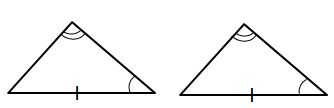#### Question 7 7. If the pictured triangles are congruent, what reason can be given?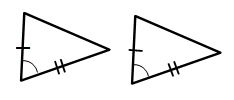#### Question 8 8. If triangle MNO is congruent to triangle PQR, CPCTC explains which of the following statements?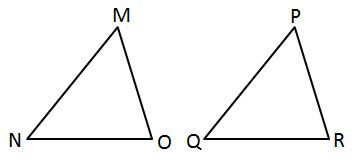#### Question 9 9. What additional information do you need to prove that triangle ABC is congruent to triangle DEF using the HL theorem?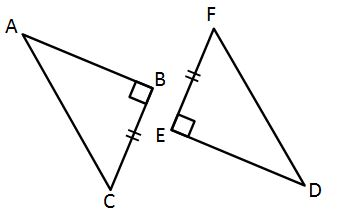#### Question 10 10. In the pictured triangle, ∠A is 137 degrees and ∠B is 28 degrees. If side b is 71 units long, approximately how long is side a?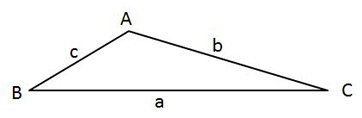### Page 3

#### Question 11 11. If the two triangles pictured below are similar, then what is x?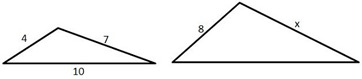#### Question 12 12. In the pictured triangle, ∠A is 98 degrees and ∠B is 12 degrees. If side a is 84 units long, approximately how long is side b?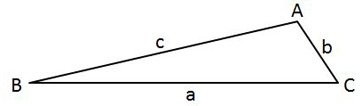#### Question 13 13. In the pictured triangle, ∠A is 81 degrees and ∠B is 67 degrees. If side a is 34 units long, approximately how long is side b?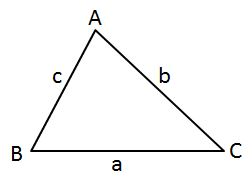### Page 4

#### Question 16 16. If triangle MNO is congruent to triangle PQR, then what is the measure of angle x?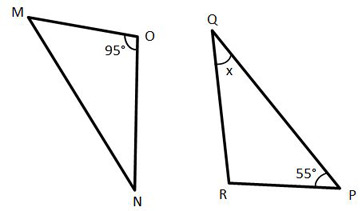#### Question 17 17. If triangle MNO is congruent to triangle STR, what must also be true?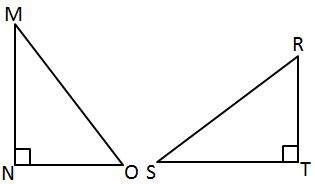#### Question 19 19. If the pictured triangles are congruent, what reason can be given?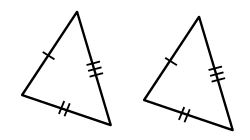#### Question 20 20. Find the measure of MN: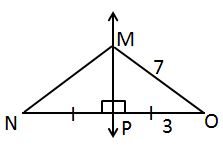### Page 5

#### Question 21 21. In the pictured triangle, side a is 23, side b is 18, and side c is 16. What is the approximate measure of ∠C? Round ∠C to the nearest whole number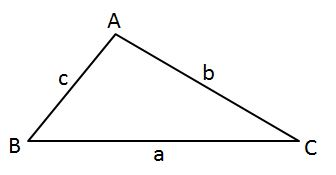#### Question 22 22. AD is a perpendicular bisector. Find the measure of AC: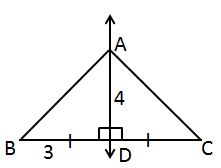#### Question 24 24. In the pictured triangle, side a is 9 and side b is 6. If ∠C is 26 degrees, then approximately how long is side c? Round the value of the cosine to the nearest whole number.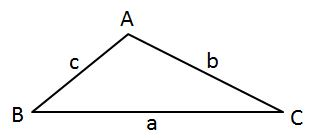#### Question 25 25. If angle a is 30 degrees and angle d is 70 degrees, what is the measure of angle b?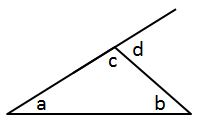### Page 6

#### Question 26 26. Find the measure of XZ: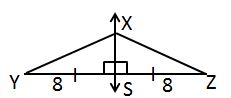#### Question 27 27. Triangle HYV and triangle AYB are similar by the AA similarity theorem. What is the value of x?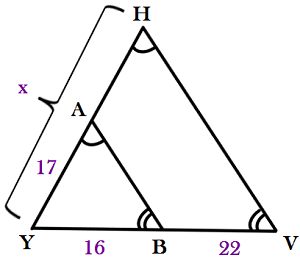#### Question 29 29. In the pictured triangle, ∠A is 58 degrees and ∠C is 63 degrees. If side a is 84 units long, approximately how long is side c?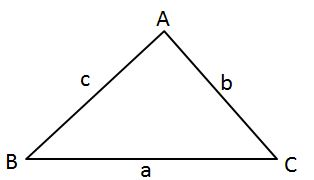#### CSET Math: Plane Euclidean Geometry Chapter Exam Instructions

Choose your answers to the questions and click 'Next' to see the next set of questions. You can skip questions if you would like and come back to them later with the yellow "Go To First Skipped Question" button. When you have completed the practice exam, a green submit button will appear. Click it to see your results. Good luck!

Support